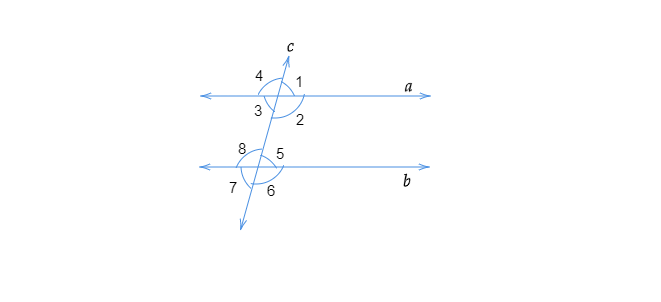$(i)$ the pairs of corresponding angles.
$(ii)$ the pairs of alternate interior angles.
$(iii)$ the pairs of interior angles on the same side of the transversal.
$(iv)$ the vertically opposite angles." ">

# In the adjoining figure, identify$(i)$ the pairs of corresponding angles.$(ii)$ the pairs of alternate interior angles.$(iii)$ the pairs of interior angles on the same side of the transversal.$(iv)$ the vertically opposite angles."

To do:

We have to identify

(i) the pairs of corresponding angles.

(ii) the pairs of alternate interior angles.

(iii) the pairs of interior angles on the same side of the transversal.

(iv the vertically opposite angles.

Solution:

(i) The pairs of corresponding angles are $\angle 1$ and $\angle 5,\ \angle 2$ and $\angle 6,\ \angle 4$ and $\angle 8,\ \angle 3$ and $\angle 7$

(ii) The pairs of alternate interior angles are $\angle 2$ and $\angle 8$; $\angle3$ and $\angle 5$

(iii) The pairs of interior angles on the same side of the transversal are $\angle 2$ and $\angle 5,\ \angle 3$ and $\angle 8$.

(iv) $\angle 1$ and $\angle 3$; $\angle 2$ and $\angle 4$; $\angle 6$ and $\angle 8$; $\angle 5$ and $\angle 7$ are the vertically opposite angles.

Updated on: 10-Oct-2022

33 Views